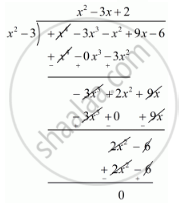Share

# Obtain All Zeros of the Polynomial F(X) = X4 − 3x3 − X2 + 9x − 6, If Two of Its Zeros Are -sqrt3 and Sqrt3 - CBSE Class 10 - Mathematics

ConceptDivision Algorithm for Polynomials

#### Question

Obtain all zeros of the polynomial f(x) = x4 − 3x3 − x2 + 9x − 6, if two of its zeros are -sqrt3 and sqrt3

#### Solution

we know that, if x = a is a zero of a polynomial, then x - a is a factor of f(x).

since -sqrt3 and sqrt3 are zeros of f(x).

Therefore

(x+sqrt3)(x-sqrt3)=x^2+sqrt3x-sqrt3x-3

= x2 - 3

x2 - 3 is a factor of f(x). Now , we divide f(x) = x4 − 3x3 − x2 + 9x − 6 by g(x) = x2 - 3 to find the other zeros of f(x).By using that division algorithm we have,

f(x) = g(x) x q(x) + r(x)

x4 − 3x3 − x2 + 9x − 6 = (x2 - 3)(x2 - 3x + 2) + 0

x4 − 3x3 − x2 + 9x − 6 = (x2 - 3)(x2 - 2x + 1x + 2)

x4 − 3x3 − x2 + 9x − 6 = (x2 - 3)[x(x - 2) - 1(x - 2)]

x4 − 3x3 − x2 + 9x − 6 = (x2 - 3)[(x - 1)(x - 2)]

x4 − 3x3 − x2 + 9x − 6 = (x - sqrt3)(x+sqrt3)(x-1)(x-2)

Hence, the zeros of the given polynomials are -sqrt3, sqrt3, +1 and +2.

Is there an error in this question or solution?

#### APPEARS IN

RD Sharma Solution for 10 Mathematics (2018 to Current)
Chapter 2: Polynomials
Ex. 2.30 | Q: 5 | Page no. 57

#### Video TutorialsVIEW ALL 

Solution Obtain All Zeros of the Polynomial F(X) = X4 − 3x3 − X2 + 9x − 6, If Two of Its Zeros Are -sqrt3 and Sqrt3 Concept: Division Algorithm for Polynomials.
S